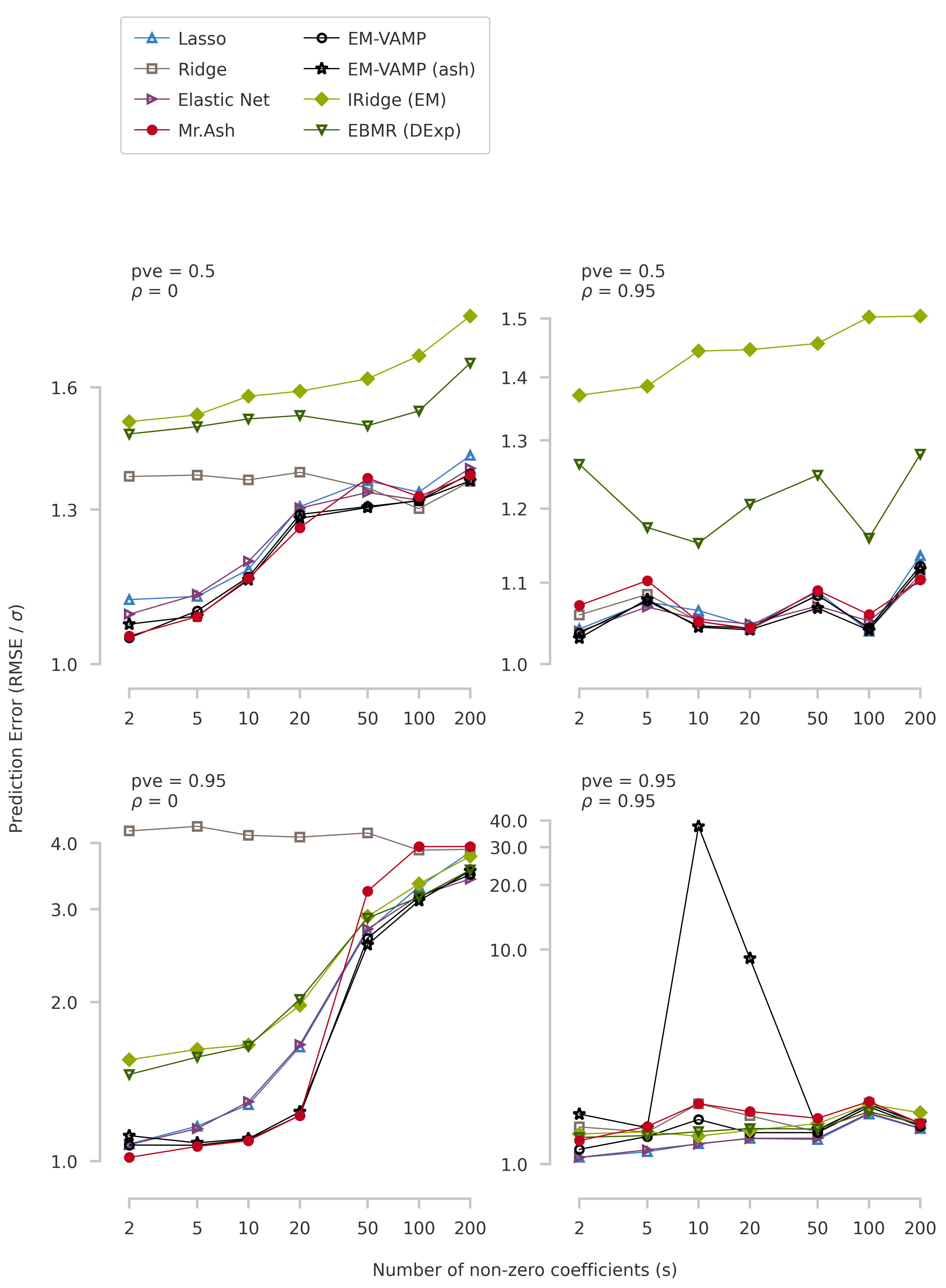Here, I compare the prediction accuracy of EM-VAMP and EM-iRidge with existing penalized regression method. The simulation scenarios were used earlier for comparing few well-known penalized regression methods.

• EM-VAMP. Proposed by Fletcher and Schniter, 2017, this algorithm combines Vector Approximate Message Passing (VAMP) and Expectation Maximization and is well suited for sparse linear regression. I used vampyre for the implementation.

• EM-VAMP (ash). Instead of a spike-and-slab prior, I use the adaptive shrinkage (ash) prior for the coefficients. See, for example, here for further details.

• EM-iRidge. Proposed by Matthew Stephens, 2020, this algorithm uses iterative ridge regression to solve linear regression where the prior of the coefficients is given by a product of two normal distributions, which has sparsity inducing properties. I implemented an Expectation Maximization algorithm for iRidge in ebmrPy.

• EBMR (DExp). Proposed by Matthew Stephens, 2020, this algorithm uses Empirical Bayes regression, where the prior variance of the coefficient depends hierarchically on another distribution.

The simulation pipeline is implemented using Dynamic Statistical Comparisons (DSC).

### Importing packages and DSC results

Non-standard packages include DSC and PyMir. The simulation repository needs to be in path for importing some of the utilities.

import pandas as pd
import numpy as np
import math
import os
import sys
import collections

srcdir = "/home/saikat/Documents/work/ebmr/simulation/eb-linreg-dsc"
sys.path.append(os.path.join(srcdir, "analysis"))
import dscrutils2py as dscrutils
import methodprops
import methodplots
import convergence_plots as convplots
import dsc_extract

import matplotlib.pyplot as plt
import matplotlib.gridspec as gridspec
from pymir import mpl_stylesheet
from pymir import mpl_utils
from pymir import pd_utils
mpl_stylesheet.banskt_presentation()


I have run the simulations using the following settings.

# Dimensions (n, p)
highdims = (100, 200)
lowdims  = (500, 200)

# fraction of non-zero predictors, sfrac = p_causal / p
sfracs   = [0.01, 0.025, 0.05, 0.1, 0.25, 0.5, 1.0]

# PVE
pve_list = [0.5, 0.95]

# rho
rho_list = [0, 0.95]

# Output directory
dsc_outdir = os.path.join(srcdir, "dsc/dsc_result")


Read the results of the simulation and store it in a DataFrame

targets = ["simulate", "simulate.dims", "simulate.se", "simulate.rho",
"simulate.sfrac", "simulate.pve", "fit", "fit.DSC_TIME", "mse.err"]
dscout = dscrutils.dscquery(dsc_outdir, targets)
dscout['score1'] = np.sqrt(dscout['mse.err'])/dscout['simulate.se']

Calling: dsc-query /home/saikat/Documents/work/ebmr/simulation/eb-linreg-dsc/dsc/dsc_result -o /tmp/Rtmp8RSKJc/file276b4c4e5568.csv --target "simulate simulate.dims simulate.se simulate.rho simulate.sfrac simulate.pve fit fit.DSC_TIME mse.err" --force
Loaded dscquery output table with 22400 rows and 12 columns.


INFO: Note: NumExpr detected 16 cores but "NUMEXPR_MAX_THREADS" not set, so enforcing safe limit of 8.
INFO: NumExpr defaulting to 8 threads.

dscout

DSC simulate simulate.dims simulate.se simulate.rho simulate.sfrac simulate.pve fit fit.DSC_TIME mse.err score1
0 1 indepgauss (500,200) 3.462448 0.00 0.010 0.50 l0learn 0.417 12.563997 1.023719
1 1 indepgauss (100,200) 0.861522 0.00 0.010 0.50 l0learn 0.872 0.654125 0.938780
2 1 indepgauss (500,200) 1.905777 0.00 0.025 0.50 l0learn 0.407 3.574130 0.992003
3 1 indepgauss (100,200) 1.249624 0.00 0.025 0.50 l0learn 0.800 1.806580 1.075596
4 1 indepgauss (500,200) 3.063747 0.00 0.050 0.50 l0learn 0.372 9.284629 0.994556
... ... ... ... ... ... ... ... ... ... ... ...
22395 20 equicorrgauss (100,200) 2.003817 0.95 0.250 0.95 mcp 0.839 10.202592 1.594033
22396 20 equicorrgauss (500,200) 0.856860 0.95 0.500 0.95 mcp 17.814 1.525528 1.441453
22397 20 equicorrgauss (100,200) 0.841918 0.95 0.500 0.95 mcp 0.866 6.398867 3.004566
22398 20 equicorrgauss (500,200) 0.964054 0.95 1.000 0.95 mcp 18.341 5.848087 2.508451
22399 20 equicorrgauss (100,200) 2.148883 0.95 1.000 0.95 mcp 0.789 15.720326 1.845092

22400 rows × 11 columns

Select the methods to be displayed in the figures.

whichmethods = [#"l0learn",
"lasso",
"ridge",
"elastic_net",
#"mcp",
#"blasso",
#"bayesb",
#"susie",
#"varbvs",
#"varbvsmix",
"mr_ash",
"em_vamp",
"em_vamp_ash",
"em_iridge",
"ebmr_lasso",
#"ebmr_ash",
]


### High dimension setting (p > n)

Simulations were performed with n = 100, p = 200. Some of the EM-VAMP optimizations did not converge. Therefore, I used median of the prediction scores (instead of mean).

highdim_condition = [f"$(simulate.dims) == '({highdims},{highdims})'"] resdf1 = pd_utils.select_dfrows(dscout, highdim_condition) methodplots.create_figure_prediction_error(whichmethods, resdf1, highdims, rho_list, pve_list, sfracs, use_median = True)### Low dimension setting (p < n) Simulations were performed with n = 500, p = 200. lowdim_condition = [f"$(simulate.dims) == '({lowdims},{lowdims})'"]
resdf2           = pd_utils.select_dfrows(dscout, lowdim_condition)

methodplots.create_figure_prediction_error(whichmethods, resdf2, lowdims,
rho_list, pve_list, sfracs, use_median = True)